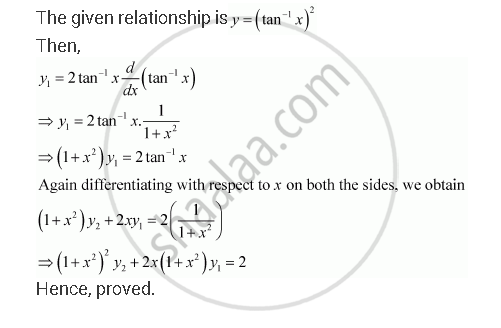Share

# If Y = (Tan–1 X)2, Show that (X2 + 1)2 Y2 + 2x (X2 + 1) Y1 = 2 - CBSE (Commerce) Class 12 - Mathematics

#### Question

If y = (tan–1 x)2, show that (x2 + 1)2 y2 + 2x (x2 + 1) y1 = 2

#### SolutionIs there an error in this question or solution?

#### APPEARS IN

NCERT Solution for Mathematics Textbook for Class 12 (2018 to Current)
Chapter 5: Continuity and Differentiability
Q: 17 | Page no. 184

#### Video TutorialsVIEW ALL 

Solution If Y = (Tan–1 X)2, Show that (X2 + 1)2 Y2 + 2x (X2 + 1) Y1 = 2 Concept: Second Order Derivative.
S